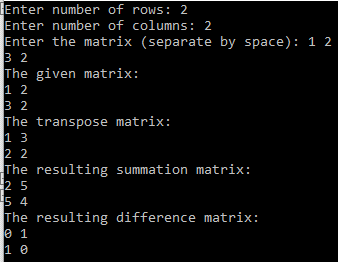# Transpose Matrix With Summation and Difference Between Main and Transpose Matrix in CHi guys , I just tried to code of transpose matrix and then summation and difference between main and that transpose matrix .
Check the screenshot I’ve attached above .

I hope you like it , and just let me know in comment if you have  any better solution .

#include<stdio.h>

int main() {
int i, j, r, c;

printf(“Enter number of rows: “);
scanf(“%d”, &r);
printf(“Enter number of columns: “);
scanf(“%d”, &c);
int a[r][c], b[c][r] , z[r][c] , x[r][c];

printf(“Enter the matrix (separate by space): “);
for ( i =0 ; i< r; i++ ) {
for ( j = 0; j < c; j++ ) {
scanf(“%d”, &a[i][j]);
}
}

printf(“The given matrix: \n”);
for ( i =0 ; i< r; i++ ) {
for ( j = 0; j < c; j++ ) {
printf(“%d “, a[i][j]);
}
printf(“\n”);
}

for ( i =0 ; i< r; i++ ) {
for ( j = 0; j < c; j++ ) {
b[j][i] = a[i][j];
}
}

printf(“The transpose matrix: \n”);
for ( i =0 ; i< c; i++ ) {
for ( j = 0; j < r; j++ ) {
printf(“%d “, b[i][j]);
}
printf(“\n”);
}

for ( i =0 ; i< r; i++ ) {
for ( j = 0; j < c; j++ ) {

z[i][j] = a[i][j] + b[i][j];
x[i][j] = a[i][j] – b[i][j];

}
}

printf(“The resulting summation matrix: \n”);
for ( i =0 ; i< r; i++ ) {
for ( j = 0; j < c; j++ ) {
printf(“%d “, z[i][j]);
}
printf(“\n”);
}

printf(“The resulting difference matrix: \n”);
for ( i =0 ; i< r; i++ ) {
for ( j = 0; j < c; j++ ) {
printf(“%d “, abs(x[i][j]));
}
printf(“\n”);
}

return 0;
}